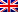# Internal quality criteria

Contenus

## Internal quality criteria

Internal quality criteria (Condorcet criterion, C criterion, category utility metric, cut measurements) generally measure the compactness of clusters using a similarity measure. It typically measures intra-cluster homogeneity, inter-cluster separability, or a combination of these two. It does not use external information alongside the data itself. Here are four internal quality criteria.

## Condorcet criterion

Another suitable approach is to apply Condorcet's solution to the classification problem. In this case, the criterion is calculated as follows:

where s (x_j, x_k) and d (x_j, x_k) measure the similarity and distance of vectors x_j and x_k.

## Criterion C

Criterion C is an extension of Condorcet's criterion and is defined as (where γ is a threshold value):

## Category utility metric

Category utility is defined as increasing the expected number of entity values that can be correctly predicted given a certain grouping. This metric is useful for problems that contain a relatively small number of nominal features each having a small cardinality.

## Cutting measures

In some cases, it is useful to represent the clustering problem as a minimal cutting problem. In such cases, the quality is measured as the ratio of the remaining weights to the total cut weights. If there is no restriction on the size of the clusters, it is easy to find the optimal value. Thus, the min-cut measurement is revised to penalize unbalanced structures.FRFRENRUES